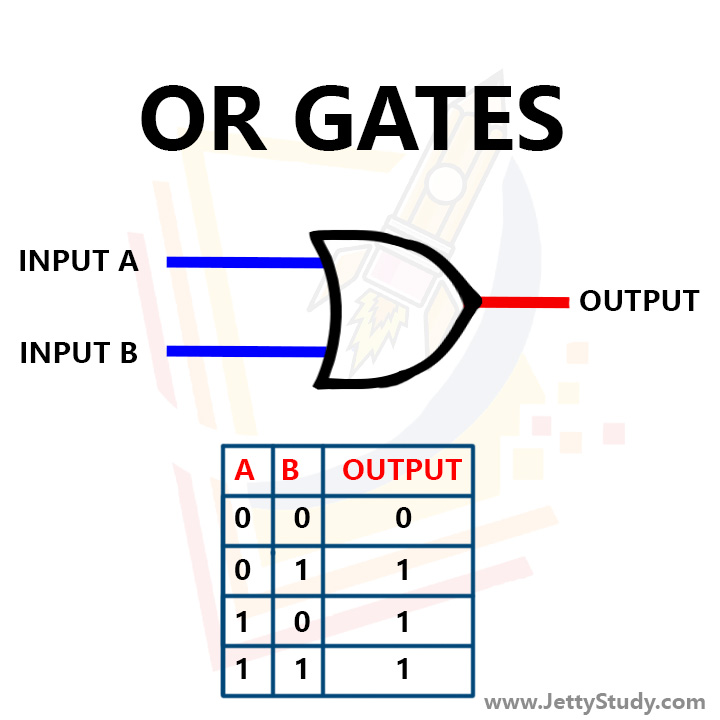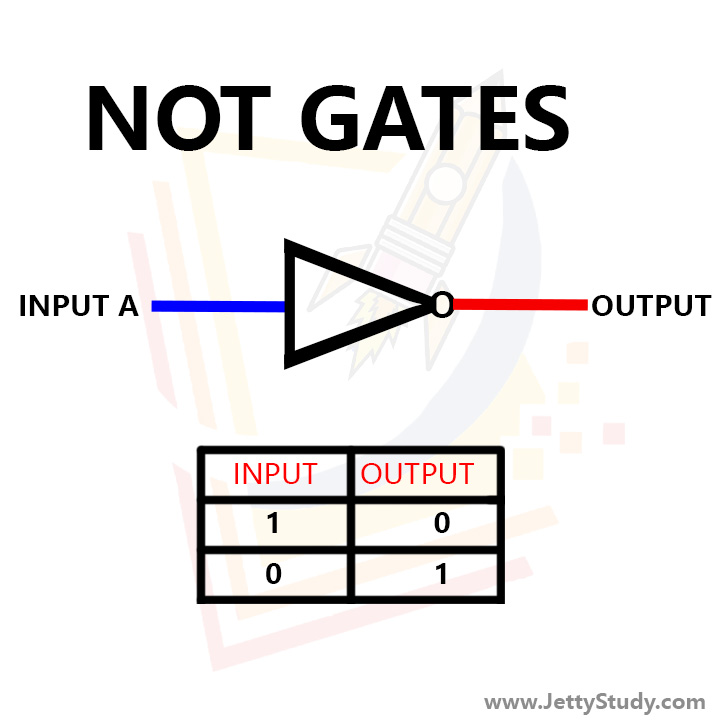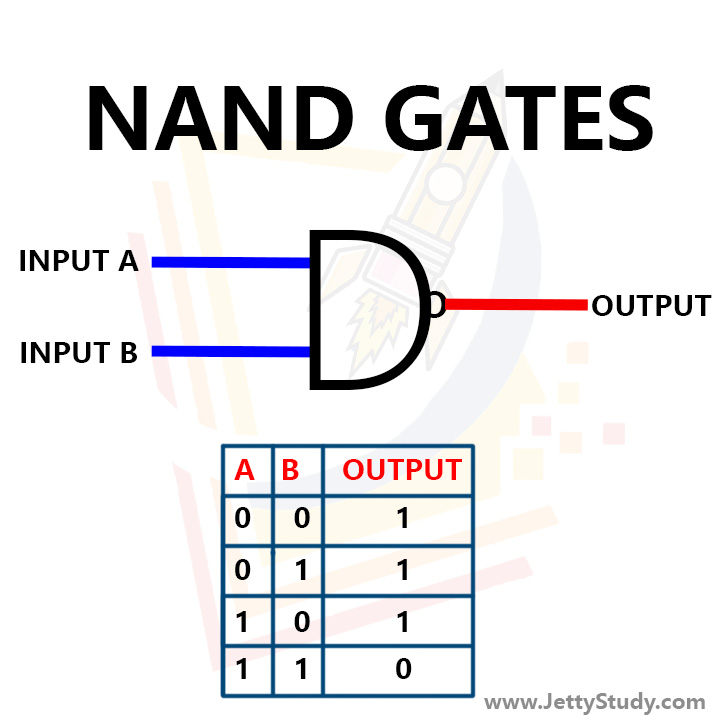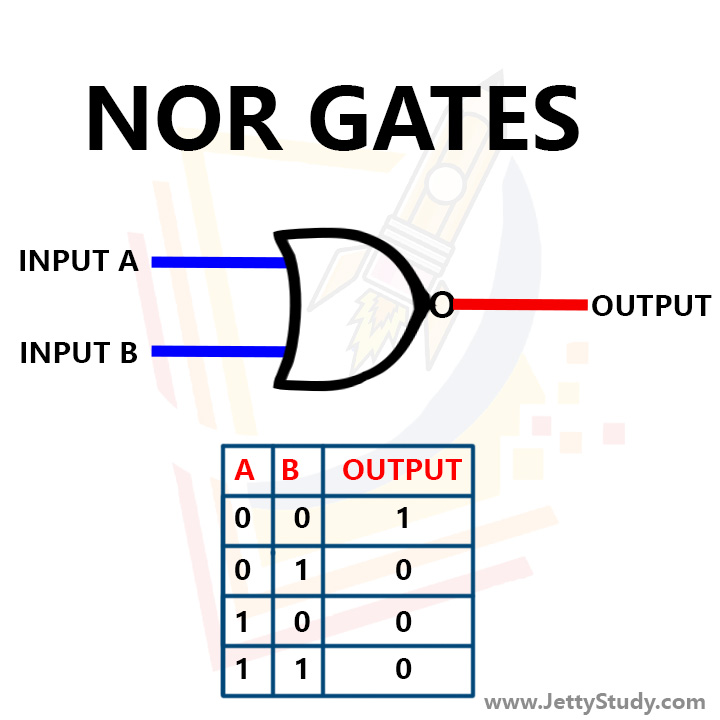# INTRODUCTION

In computer organization, combinatorial blocks refer to the logic gates that are used to perform various operations on the input data. These gates are the building blocks of digital circuits and are used to create combinatorial circuits that perform specific functions, such as logical operations, arithmetic operations, and data manipulation. These blocks are used to create the control unit and the arithmetic logic unit (ALU) in a computer's central processing unit (CPU).

AND GATE : The AND gate is a digital logic gate that performs a logical AND operation on its two or more inputs. The output of an AND gate is 1 only if all of its inputs are 1, otherwise the output is 0. It is represented by the symbol '&' in Boolean algebra. AND gate is widely used in digital circuits for a variety of applications.OR GATE : The OR gate is a digital logic gate that performs a logical OR operation on its two or more inputs. The output of an OR gate is 1 if any of its inputs is 1, otherwise the output is 0. It is represented by the symbol '|' in Boolean algebra. OR gate is widely used in digital circuits for a variety of applications.NOT GATE : The NOT gate is a digital logic gate that performs a logical NOT operation on its input. The output of a NOT gate is the opposite of its input. If the input is 0, the output is 1 and if the input is 1, the output is 0. It is represented by the symbol '!' in Boolean algebra. NOT gate is widely used in digital circuits for a variety of applications.NAND GATE : The NAND gate is a digital logic gate that performs a logical NAND operation on its two or more inputs. The output of a NAND gate is 1 only if all of its inputs are 0, otherwise the output is 0. It is represented by the symbol '&!' in Boolean algebra. NAND gate is widely used in digital circuits for a variety of applications.NOR GATE : The NOR gate is a digital logic gate that performs a logical NOR operation on its two or more inputs. The output of a NOR gate is 1 only if all of its inputs are 0, otherwise the output is 0. It is represented by the symbol '|!' in Boolean algebra. NOR gate is widely used in digital circuits for a variety of applications.# CONCLUSION

Combinatorial blocks are an essential component of computer organization. They are used to perform various operations on the input data and are the building blocks of digital circuits. These gates, such as AND, OR, NOT, NAND, and NOR, are used to create combinatorial circuits that perform specific functions, such as logical operations, arithmetic operations, and data manipulation. These blocks are used to create the control unit and the arithmetic logic unit (ALU) in a computer's central processing unit (CPU) and play a crucial role in the functioning of a computer.